# VAT Returns

I am looking at the VAT returns, and I am struggling to understand why some VAT adjustments are added and some are deducted when calculating boxes 6 and 7? How would you know whether to add or deduct the VAT amount or the gross amount?

Could someone explain- examples below.

First example- box 6 has been calculated as- net sales- VAT on adjustment (8456-250= 8206)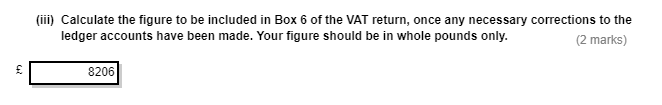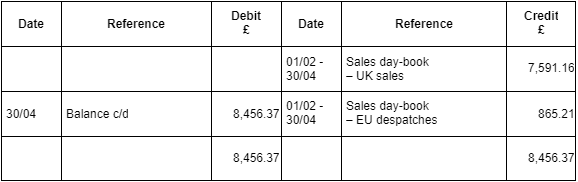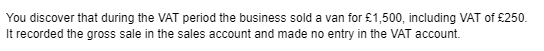Second example- box 6 has been calculated as- net sales + gross amount of VAT adjustment. (389184+573.80)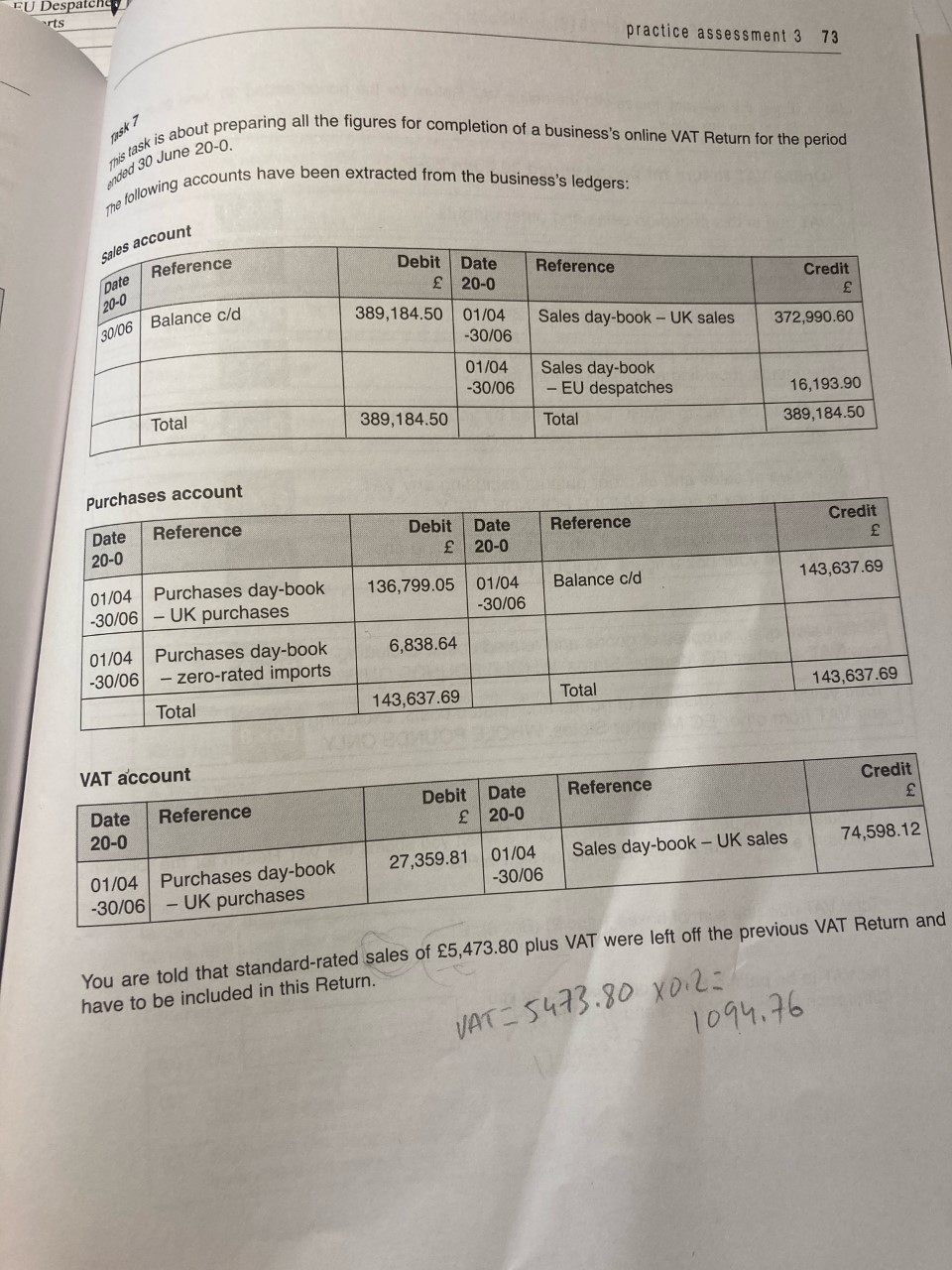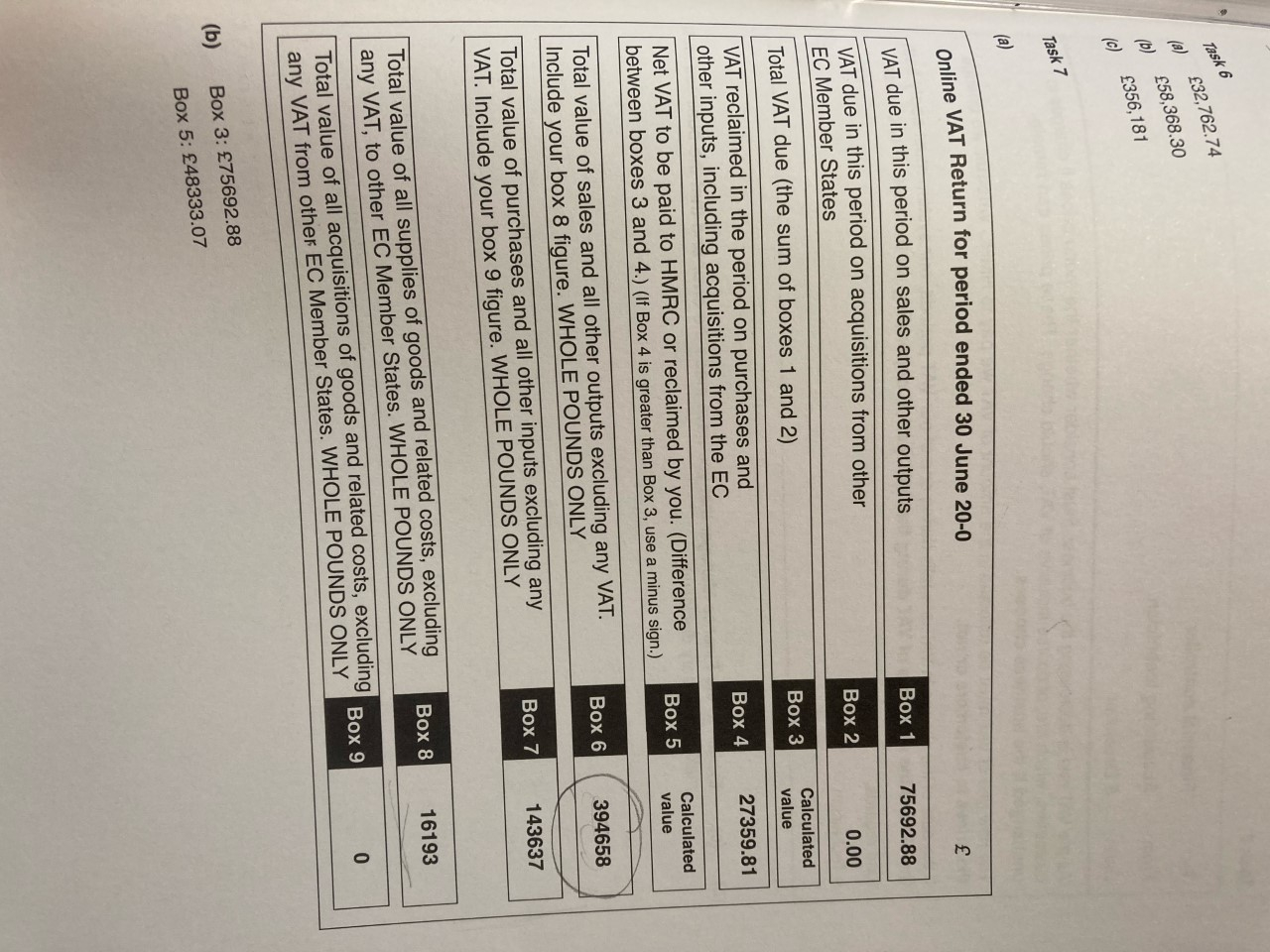## Comments

• In the example 1, the second line mentions gross sale amount of car(1500) was added to the sales account. Which means 8457.37 has 1500(including vat of 250). the sales account needs to be corrected to net value.
So deduct vat amount 250 from 8457.37 to show only the net sales.

In example 2, vat amount value of 1094.76 is included in box 6. I could not figure out the reason.

Chan
• Hi,
Checked again example 2.
box 6 shows the correct figure. In shows only the net sales.
Sales account balance 389184.50+5473.80(prev return omitted net sales) = 394658

Chan
• Right, so for example 1- because the gross amount was added to the sales amount, the figure of 8456.37 includes a mixture of gross and net sales when it should ONLY include net sales? The way to reverse/adust this is through ensuring that the gross amount that has been included is by deducting VAT to leave us with only net sales?

Example 2- The net sales of 5473.80 were omitted from the amount, therefore this now needs to be included. So just like we would have added the VAT to box 1, we now need to include the net sales in the overall amount?
Sign In or Register to comment.